2.1Theory

2.1.1Memristor: The Fourth Element

The four fundamental circuit variables are electric current, voltage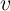, charge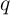, and flux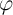, where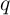and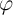are defined as the time integrals ofand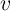, respectively .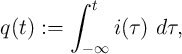(2.1)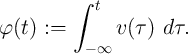(2.2)

The three conventional two-terminal basic circuit elements resistor, capacitor, and inductor are defined in terms of the constitutive relationships between two of these four variables as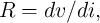(2.3)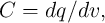(2.4)(2.5)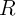,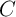, and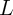are the resistance, capacitance, and inductance, respectively. Eq. 2.1-Eq. 2.5 express five from six possible relations between the fundamental circuit variables (Fig. 2.1). For the sake of completeness, Leon Chua postulated the existence of a fourth fundamental two-terminal circuit element called memristor (memory resistor)  characterized by a constitutive relationship between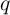and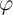in which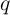and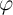are not necessarily accessible to any physical interpretation . The constitutive relation of charge-controlled and flux-controlled memristors are obtained as Eq. 2.6 and Eq. 2.7, respectively .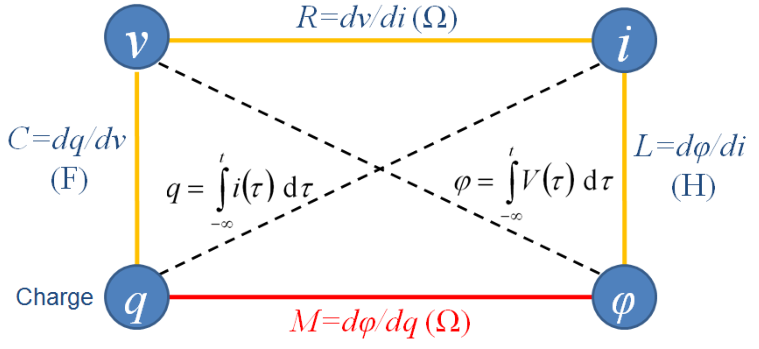Figure 2.1.: Chua’s symmetry argument.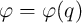(2.6)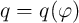(2.7)

By taking the time derivatives we obtain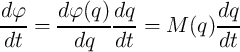(2.8)

and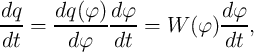(2.9)

where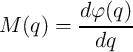(2.10)

and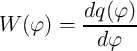(2.11)

are the memristance and the memductance of the memristors as a function of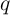and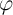, respectively.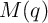and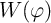have the units of Ohms (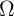) and Siemens (S) as according to the definitions (Eq. 2.1 and Eq. 2.2) we have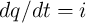andand thus we can simplify Eq. 2.8 and Eq. 2.9 to Eq. 2.12 and Eq. 2.13, respectively.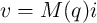(2.12)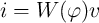(2.13)

In fact, a charge (flux)-controlled memristor is characterized by a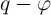curve and its memristance (memductance) at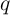(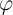) is equal to the slope of the curve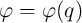(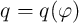). A device with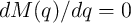(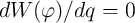) is just a linear resistor (conductor), while if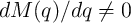(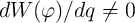) the device operates like a variable resistor (conductor) and exhibits memristive behavior. In Chapter 6 we will see that memristors with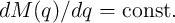(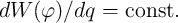) are suited for a new charge (flux)-based memristive sensing scheme.

The memristor acts as a programmable resistor since its electrical resistance depends on the time integral of the applied current/voltage. Ideally, when the power is turned off (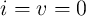), the memristor preserves its resistance forever, as the values of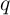and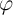are left unchanged . Therefore, it records the historic profile of the applied current or voltage in the memristance/memductance which can be revealed instantaneously by measuring its electrical resistance. This is a unique property of memristors which cannot be realized by electric circuits combining resistors, capacitors, and inductors. The most straightforward application of a memristor is non-volatile memory either as an analog (continuously tunable or multilevel) memory or as a digital switch, depending on the physical operating mechanisms of the resistance switching in the memristive device. In general, one can say a memristor operates as an analog device in a low-voltage regime, while under large voltages it operates as a digital switch between two states, characterized by low and high resistances corresponding to the minimum and the maximum achievable resistance values limited by the physical properties of the device.

Since the memristor is a passive device, its current-voltage characteristic exhibits a hysteresis loop pinched at the origin and confined to the first and the third quadrants . In fact, when the current (voltage) applied to the memristor goes to zero at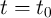, the memristor acts as an ordinary resistor (conductor) with a finite resistance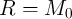(conductance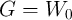) and thus the voltage (current) of the memristor goes to zero as well. Therefore, the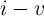curve passes through the origin and pinches the memristor hysteresis loop. It is clear that the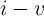characteristics of any nonlinear resistor (e.g. memristor) cannot be a straight line that passes through the origin, otherwise it is a linear resistor. Four decades after Chua’s seminal paper () on memristor, he recently has shown that all forms of two-terminal non-volatile memories based on resistance switching can be classified as memristor since they demonstrate memristor fingerprint characterized as a pinched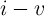hysteresis loop [71, 72].

2.1.2Memristive Systems

The definition of a memristor can be extended to a (passive two-terminal) memristive system  described by two coupled equations as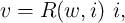(2.14)

called State-dependent Ohm’s law  and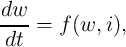(2.15)

called State equation , where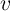is the voltage across the device,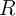represents the generalized (nonlinear) resistance of the device,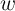denotes a state variable which can be a vector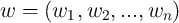,is the current through the device, andexpresses the functional dependence of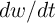on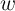and. The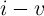curve passes through the origin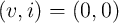as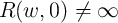. As we will see later, the state variable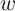can describe physically reasonable device parameters and thus this general definition of a memristive system can be successfully utilized to model various memristive devices with different operating mechanisms.